$$\newcommand{\dint}{\mathrm{d}} \newcommand{\vphi}{\boldsymbol{\phi}} \newcommand{\vpi}{\boldsymbol{\pi}} \newcommand{\vpsi}{\boldsymbol{\psi}} \newcommand{\vomg}{\boldsymbol{\omega}} \newcommand{\vsigma}{\boldsymbol{\sigma}} \newcommand{\vzeta}{\boldsymbol{\zeta}} \renewcommand{\vx}{\mathbf{x}} \renewcommand{\vy}{\mathbf{y}} \renewcommand{\vz}{\mathbf{z}} \renewcommand{\vh}{\mathbf{h}} \renewcommand{\b}{\mathbf} \renewcommand{\vec}{\mathrm{vec}} \newcommand{\vecemph}{\mathrm{vec}} \newcommand{\mvn}{\mathcal{MN}} \newcommand{\G}{\mathcal{G}} \newcommand{\M}{\mathcal{M}} \newcommand{\N}{\mathcal{N}} \newcommand{\S}{\mathcal{S}} \newcommand{\diag}{\mathrm{diag}(#1)} \newcommand{\diagemph}{\mathrm{diag}(#1)} \newcommand{\tr}{\text{tr}(#1)} \renewcommand{\C}{\mathbb{C}} \renewcommand{\R}{\mathbb{R}} \renewcommand{\E}{\mathbb{E}} \newcommand{\D}{\mathcal{D}} \newcommand{\inner}{\langle #1 \rangle} \newcommand{\innerbig}{\left \langle #1 \right \rangle} \newcommand{\abs}{\lvert #1 \rvert} \newcommand{\norm}{\lVert #1 \rVert} \newcommand{\two}{\mathrm{II}} \newcommand{\GL}{\mathrm{GL}} \newcommand{\Id}{\mathrm{Id}} \newcommand{\grad}{\mathrm{grad} \, #1} \newcommand{\gradat}{\mathrm{grad} \, #1 \, \vert_{#2}} \newcommand{\Hess}{\mathrm{Hess} \, #1} \newcommand{\T}{\text{T}} \newcommand{\dim}{\mathrm{dim} \, #1} \newcommand{\partder}{\frac{\partial #1}{\partial #2}} \newcommand{\rank}{\mathrm{rank} \, #1}$$

# Unsupervised Classification

## Introduction

Unsupervised classification (also called clustering) is the problem of classifying a dataset without any labels for training. An unsupervised learning algorithm is supposed to find tendencies, similarities between certain feature vectors. The goal is to group data within clusters, such that the members of one cluster are as similar as possible while being as different as possible from other clusters members.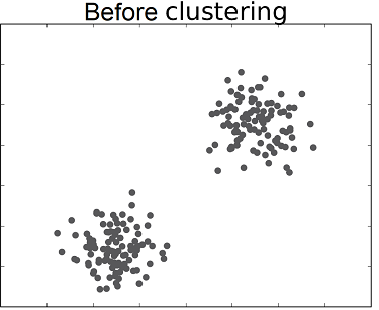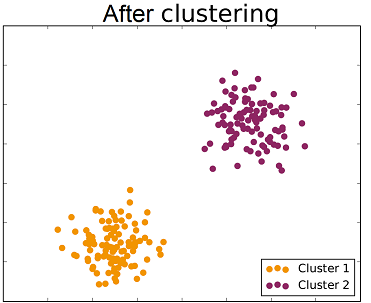The following video shows an application of clustering to unsupervised sorting of objects by a robotics system.

## K-means Algorithm

The K-means clustering algorithm principle is the following. Starting from a given dataset, the space is partitioned by centroids in the $N$ dimensional space. These centroids are simply points in this space. A data point belongs to the class represented by the centroid that is closest to itself according to the norm used (usually the euclidean norm). Hence, there should be as many centroids as the number of desired classes. The successive steps executed by $K$-means are the following.

• Centroids initialization: The $K$-means algorithm works in an iterative fashion. The first step consists in initializing the centroids. If we want to classify the data within $K$ classes, then $K$ points (with the same dimension as the feature vectors) must be introduced. There exist different methods to position such points, one of them is to choose $K$ random points among the dataset and to let the initial centroids be these points.

• Classes allocation: Once centroids are defined, the next step is to label each point according to the current centroids. Hence, for each point, we must compute its distance to each centroid and label it with the class corresponding to the closest one.

• Centroids updating: Once every point in the dataset has got a label, centroids are updated. For each one of the current classes, the mean vector is computed and the new value of the class centroid is set equal to this mean vector.

The class allocation and centroid update steps are repeated successively until there is no more evolution in both steps. Once the process is over, data are classified. The following figures show the different steps of $K$-means clustering on a two dimensional dataset with two classes.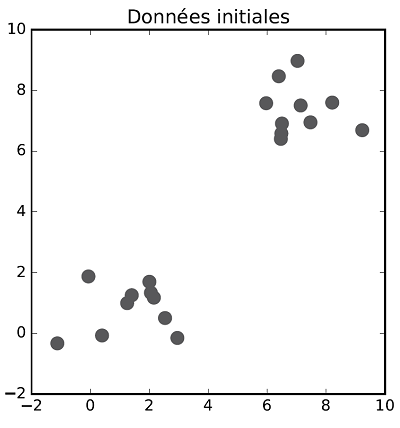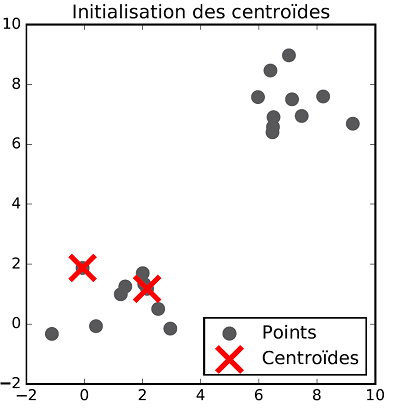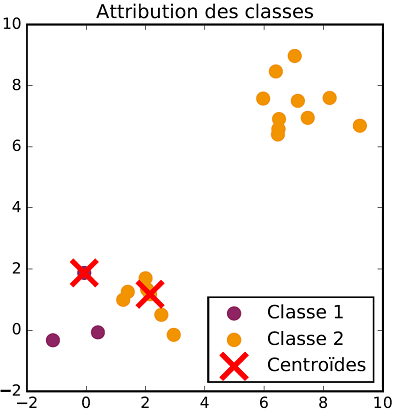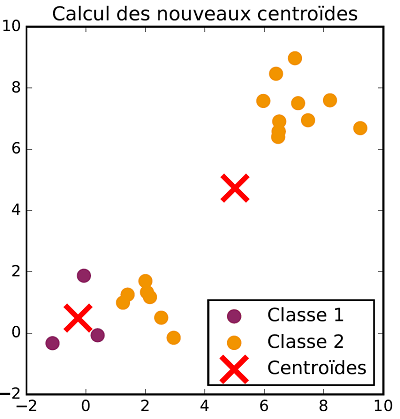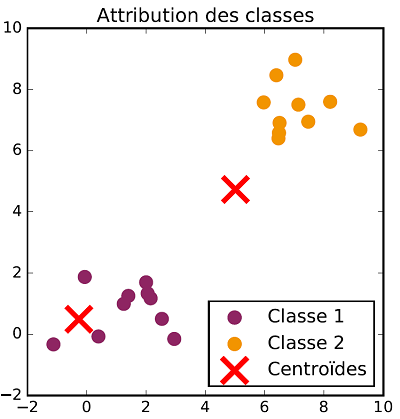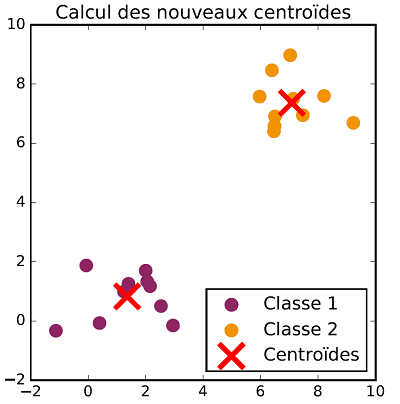Exercise[$K$-means clustering implementation]

• Implement the $K$-means clustering algorithm on the Iris dataset without using the labels (Labels can be used to check the clustering results).

• Do the same thing with the "Wine" dataset, which can be found at this link

## References

1. Franck Rosenblatt (1962), Principles of neurodynamics: perceptrons and the theory of brain mechanisms